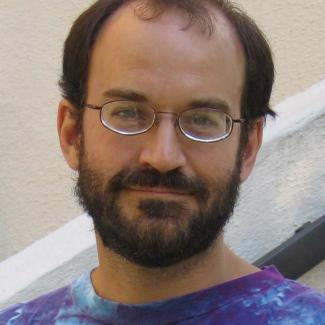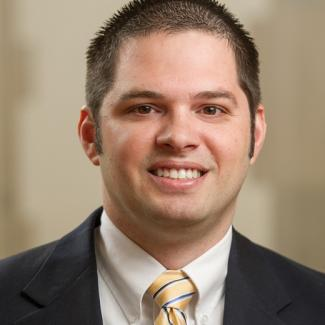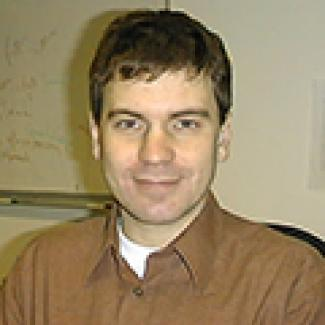Polynomial equations and inequalities are ubiquitous in the mathematical sciences and their applications. This workshop will focus on recent algorithmic advances, both numeric and symbolic, on novel domains of application, and on fundamental issues of complexity in algebraic geometry. One recent advance concerns Smale's 17th problem which asks for the solution of polynomial equations in polynomial time in the average case. Understanding and solving systems of sparse polynomials (fewnomials) offers challenging problems. The real τ-conjecture (on the number of real roots of a sum of products of sparse polynomials) establishes a fascinating new link between real algebraic geometry and fundamental complexity lower bound questions. Another topic for this workshop is convex algebraic geometry, which fuses convex optimization theory and real algebraic geometry. We will also discuss current software tools such as Bertini, Singular, SAGE, and Macaulay2, and how these may aid researchers in the CS community.

Enquiries may be sent to the organizers workshop_alggeom2 [at] lists.simons.berkeley.edu (at this address.)

Chairs/OrganizersFrank Sottile (Texas A&M University; chair)Jonathan Hauenstein (University of Notre Dame)Pascal Koiran (École Normale Supérieure de Lyon)
Invited Participants